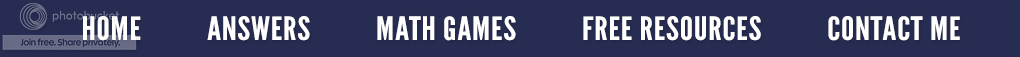### The Eleventh Hour?

The mathematician magician is still here, sharing her tricks.  This week it is the elevens.  Before we demonstrate the trick, I have to get on my soap box for just a moment.  In my humble opinion, all students should know their times tables through 12 even though the Common Core Standard for third grade says through 10 x 10.  Remember, Common Core is the minimum or base line of what is to be learned.  In Algebra, I insist that my students know the doubles through 25 x 25 and the square roots of those answers up to 625.  It saves so much time when we are working with polynomials.

Now to our our amazing mathematical "trick".  Let's look at the problem below which is 231 x 11.

First we write the problem vertically. Next, we bring down the number in the ones place which in this case is a one. Now we add the digits in the ones and tens place which is 3 + 1 and get the sum of four which is brought down into the answer.

Moving over to the hundreds place, we add that digit with the digit in the tens place 2 + 3 and get an answer of five which we bring down.  Finally, we bring down the digit in the hundreds place which is a two.  The answer to 231 x 11 is 2,541.

Now try 452 x 11 in your head.  Did you get 4,972?  Let's try one more.  This time multiply 614 by 11.  I'm waiting......  Is your answer 6,754?

Now it is time to make this process a little more difficult.  What happens if we have to regroup or carry in one of these multiplication problems?

We will multiply 784 by 11.  Notice that we start as we did before by just bringing down the number in the ones place.  Next, we add 8 + 4 and get a sum of 12.  We write down the 2 but carry or regroup the one.  We now add 7 + 8 which is 15 and then add in the 1 we are carrying.  That makes 16.  We bring down the 6 but carry the 1 over.  We have a 7 in the hundreds place, but must add in the one we are carrying to get a sum of 8.  Thus our answer is 8,624.

Let's see if you can do these without paper or pencil.  965 x 11   768 x 11    859 x 11   After working the problems in your head, write down your answers and check them with a calculator.  Try making up some four and five digit problems because this is a non-threatening way to have your students practice their multiplication facts.  Have fun!